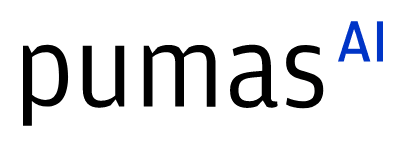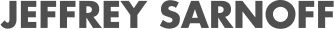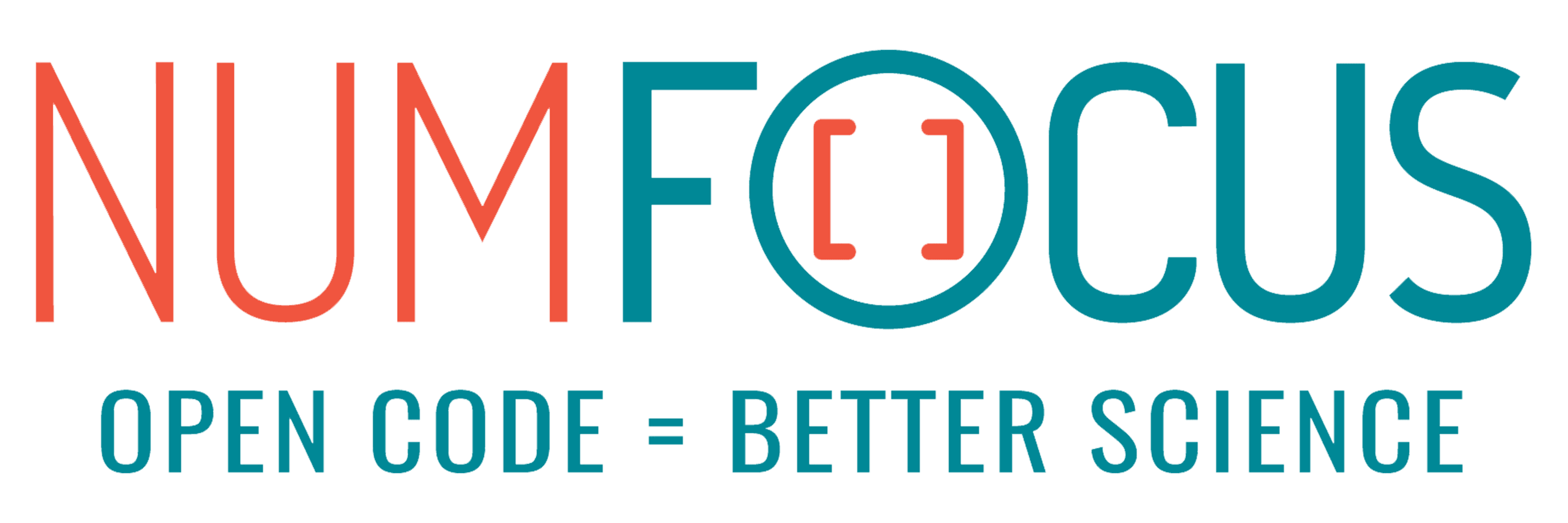# UDEs for parameter estimation in Systems Biology

07/27/2023, 7:40 PM7:50 PM UTC
32-D463 (Star)

#### Abstract:

Neural universal differential equations (UDEs) are one of the comparatively new concepts combining aspects of purely knowledge-based mechanistic models with black-box and data-based approaches. We explore the applicability of UDEs for parameter estimation in the context of systems biology. For this, we consider different levels of noise, data sparsity, and prior knowledge about the underlying system on a diverse set of problems.

#### Description:

There are two common paradigms for mathematical modelling of dynamics in life science: Knowledge-based mechanistic models like systems of ordinary differential equations or black-box approaches such as neural networks. Over the last years, methods for combining these concepts, sometimes called hybrid models, gained increasing attention in various fields of science. Neural universal differential equations (UDEs) represent one of the most prominent ideas. UDEs describe the dynamics of a system in the form of an ordinary differential equation (ODE), summing over a knowledge-based mechanistic term and a neural network-based universal approximator term. Since mechanistic parameters can jointly be optimized with the parameters of the neural network, UDEs raise hope to solve both forward as well as inverse problems with arbitrary levels of prior knowledge. Using SciMLs DiffEqFlux.jl, we explore the applicability of UDEs for parameter estimation in the context of systems biology. For this, we consider different levels of noise, data sparsity, and prior knowledge about the underlying system on problems described by ordinary differential equations. The setting of systems biology introduces difficulties like observable mappings, high-dimensional mechanistic parameter spaces and stiffness. We integrate ideas from conventional mechanistic modeling with ideas from neural network training to overcome these challenges and balance the contributions from knowledge-based mechanistic models and data-driven neural network components.